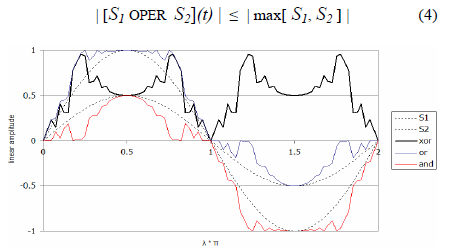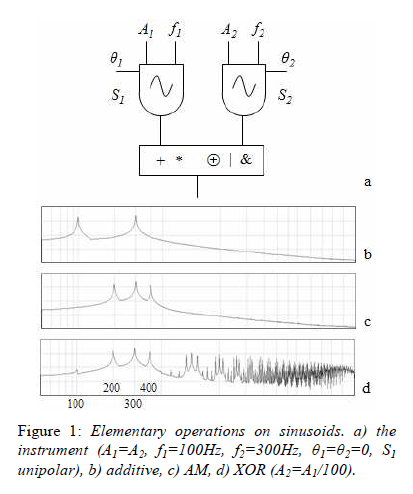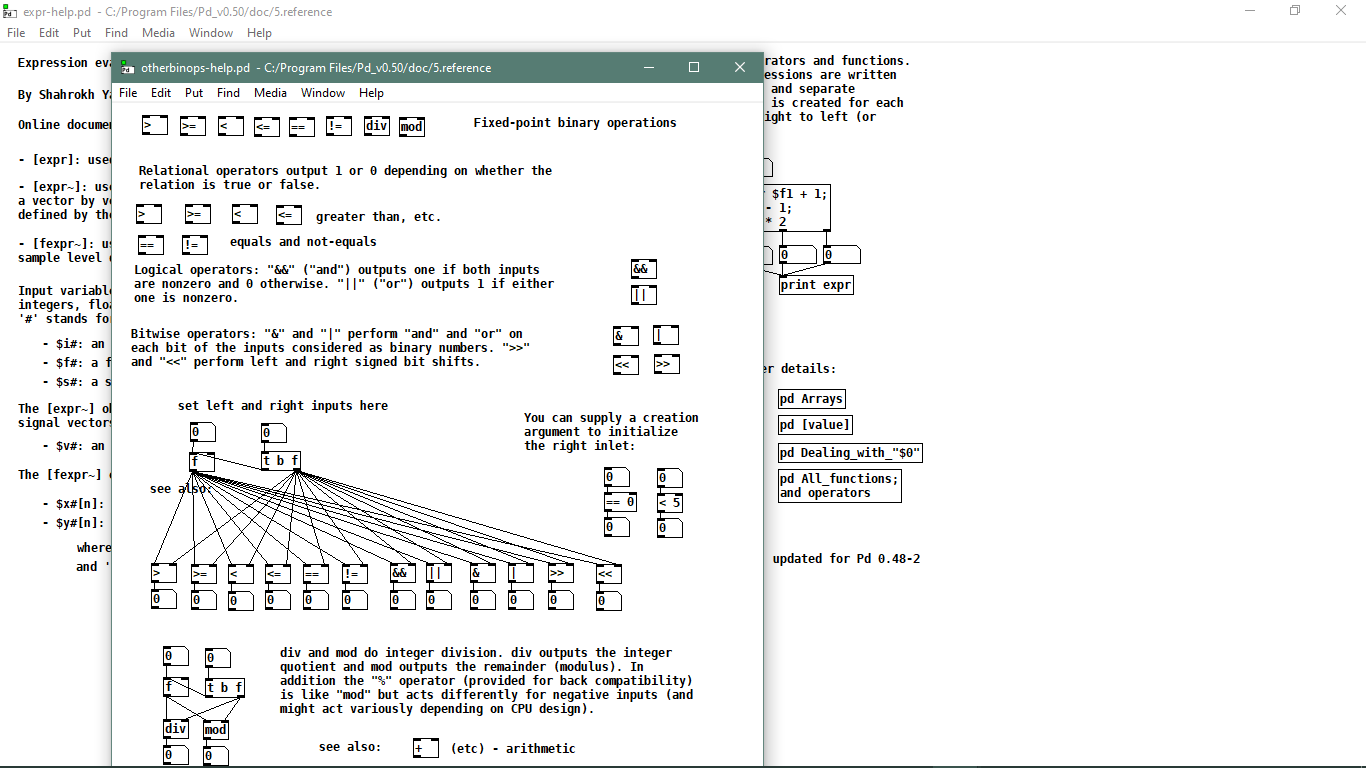• ### Converting audio signals to binary with no externals ?

How do i convert an audio signal to binary without using bitsplit~ from creb external in Pure Data ?. Do i need to use snapshot~ in combination with something else or there is a better way ? I was thinking about doing signal quantification using snapshot~ and then converting the decimal magnitude to binary. Is this the right way or is something else that i miss ? Many thanks.

• Posts 24 | Views 3414
• what do you mean? Audio is already in binary floating-point.. do you want to print every binary place-value of every single sample somewhere? Or could it be every 64 samples? (in which case I would go the snapshot~ route)

• @seb-harmonik.ar Yes i need somehow to extract all the binary digits from an audio signal for every single sample. The idea is to then apply bitwise synthesis to the binary signal and converting back to audio. So i can have a XOR gate performing bitwise operation on 2 binary vectors from 2 audio sources.

• @Boran Robert I copy this from another thread as a thought: "...plus in general, it's just never a real good idea to try to do audio signal processing with control signals (except for stuff like LFO's PWMS or other 'slow' modulations perhaps)..."
https://forum.pdpatchrepo.info/topic/7906/control-as-signal-generator/2

• @Boran-Robert I try using snapshot~ but it is not ok. It is not stable and it gives me negative numbers. Negative numbers from decimal to binary requires additional conversion to 2's complement which i don't want. Sign magnitude is not good because i will have -0 and 0 for representing the same zero.

• @Jona Yes. Snapshot~ converts it to control signal. Thank you i need to look into it.

• @Boran Robert and the audio signal is always between -1 and 1, just add [+ 1] and [/ 2] (if you want to have them between 0 and 1)...

• @Jona Yes. You are right. Thanks.

• @Boran-Robert you can use tabwrite~, so you'll have every samples.

My music on bandcamp : https://ilpleut.bandcamp.com/

• @Il-pleut Thanks.

• [snapshot~] will only give you the last value of the previous sample block, not good for any operation on all samples. Why don't you try [expr~]. For XOR you can do this `[expr~ \$v1^\$v2]`
If you want a specific number of bits (say 8-bit), add 1 to a bipolar signal and multiply it by half the resolution. For example for 8-bit do this:

``````[osc~]
|
[+~ 1]
|
[*~ 127.5]
|
[expr~ int(\$v1)^int(\$v2)]
``````

or do everything in [expr~] like this:

``````[osc~]
|
[expr~ int((\$v1+1)*127.5)^int((\$v2+1)*127.5)]
``````

That should work

• @alexandros Thank you very much. I need to further test this ideas. So more precisely i want to make bitwise XOR modulation on two sine waves. The problem is i don't now what i am missing. Another problem with "&" and "|" operations are that i need them as inputs to be already in binary form. So this operations "&" and "|" don't work with [expr~] only [expr]. But yes i would like to use [expr~] because converting the signals to binary form and then back to signal is not easy. Also for the carry oscillator i was thinking to use unipolar signal instead of bipolar.

``````[osc~ 300]  <- carry                         [osc~ 100] <- modulator
|                                            |
[expr~ here i need to make bitwise XOR,AND,OR]
|
[dac~ 1 2]
``````

so actually i try to make bitwise XOR like this :

``````[osc~ 300]                        [osc~ 100]
|                                 |
[expr~ ((\$v1 | \$v2) - (\$v1 & \$v2))]    XOR = (A OR B) - (A AND B)
|
[dac~ 1 2]
``````

This idea works for binary numbers :

``````         
|              |
[expr \$f1 & \$f2]
|
[output]

                           
|                                |
[expr ((\$f1 | \$f2) - (\$f1 & \$f2))]
|
[output]

         
|              |
[expr \$f1 | \$f2]
|
[output]

``````
• @Boran-Robert ? for & but bitwise XOR ( ^ ) seems to work...... I put it twice here just to check......

``expr~ ((\$v1 ^ \$v2) ^ -(\$v1 + \$v2))```
• I think we should clarify further what your intentions are.. Are you keeping the audio signals as floating point numbers in the range of 0 to 1 or 0 to 2? Do you want to XOR the floating point numbers or only their "integer" parts? If you XOR the raw binary representation of 2 floating point numbers I feel like the results would be way more unpredictable than in fixed point/integer because of XOR ing the exponents.. it makes my head hurt just to think about the results of that and I doubt you could do it in pd vanilla anyhow..

I think what you might want is to multiply both numbers before doing your expr~ (for instance to be in the range of 0 to (2^24 - 1) in order to get all of the significand part) since expr~ only does binops on integers/rounds to the nearest integer, then scale back into your audio range afterward (as in @alexandros' example)

• @Boran-Robert what do you mean "already in binary form"? All numbers/audio are already in binary form internally, but when you type them in number boxes or have them displayed or printed they are converted to/from decimal.. so in your example with binary numbers those numbers are actually decimal numbers if you would type them into boxes in pd, and indeed if you run your example with trying to use binary numbers you will see that the output has non-binary digits

• @Boran-Robert It is always great to see @seb-harmonik.ar and @alexandros contributing to the audio discussion on this forum.
We can learn....... rather than fumbling in the dark...!
David.

• @seb-harmonik.ar , @whale-av Many thanks for help. So what i want to do is this :

i want to have 2 oscillators (both sine waves. one a carry oscillator and the other one a modulator) with different frequency. And i want their audio signal to be processed in [expr~ ] using bitwise XOR modulation. This is different from [+~] , [-~] , [*~] , [/~]. This paper describes this in detail.

dafx08_13.pdf

Here is a plot of the waveform result using bitwise XOR (from the paper above) :Here is a schematic view of the oscillator structure :
So to control both oscillators i need to control the theta (phase) , f (frequency) and A (linear amplitude). Also S1 is unipolar. So the idea is that i can use the basic operations on the audio signals like (addition,multiplication,bitwise OR,bitwise AND) or i can use something different (bitwise XOR). So i tried to make somehow the bitwise XOR using a formula : bitwise XOR equivalent <- (S1 | S2) - (S1 & S2) (not working). The bitwise operations like "&" and "|" works for [expr] not [expr~].Also i just found this about [expr~ ] object so maybe i am missing something or i am wrong
What is this operator "^" it is bitwise XOR ? or exponential ? or something else ?Thanks to all a lot for the help. I also tried what @alexandros was talking about and it is almost working. But the waveform is really off so i need to learn more about this idea.

• @Boran Robert i think & and | do work with expr~ and ^ is XOR, see this example for & and | in use: bitshift_sound.pd
here is a list with all operators: